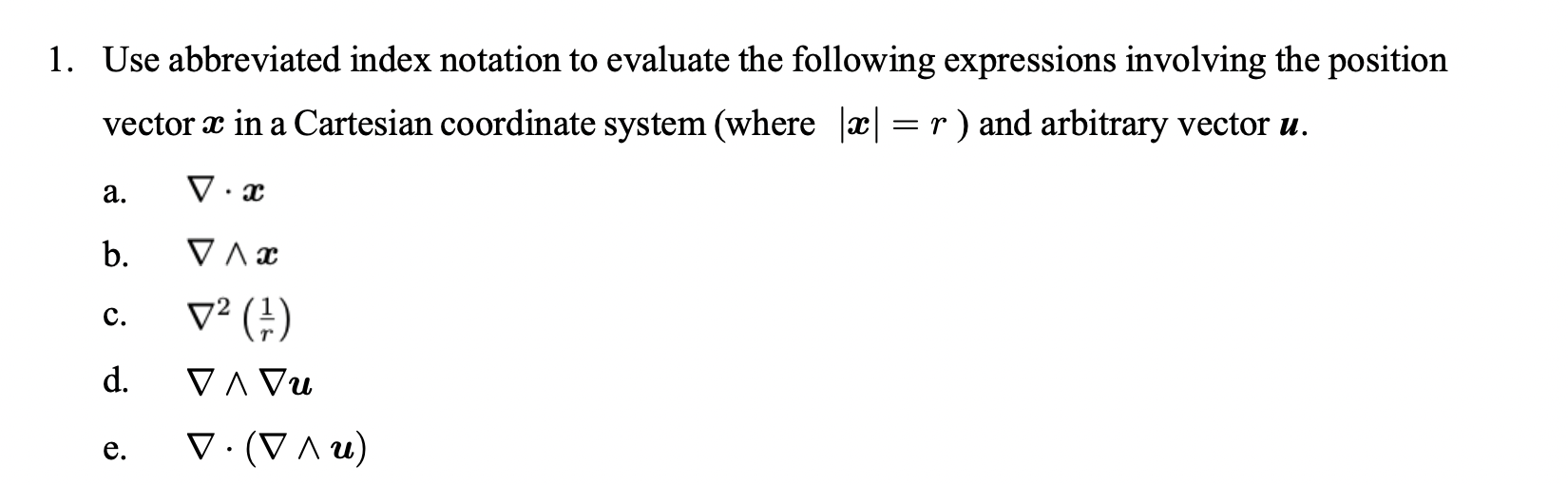Home / Expert Answers / Chemical Engineering / 1-use-abbreviated-index-notation-to-evaluate-the-following-expressions-involving-the-position-vec-pa693

# (Solved): 1. Use abbreviated index notation to evaluate the following expressions involving the position vec ...1. Use abbreviated index notation to evaluate the following expressions involving the position vector $$\boldsymbol{x}$$ in a Cartesian coordinate system (where $$|\boldsymbol{x}|=r$$ ) and arbitrary vector $$\boldsymbol{u}$$. a. $$\nabla \cdot \boldsymbol{x}$$ b. $$\nabla \wedge \boldsymbol{x}$$ c. $$\nabla^{2}\left(\frac{1}{r}\right)$$ d. $$\nabla \wedge \nabla \boldsymbol{u}$$ e. $$\nabla \cdot(\nabla \wedge \boldsymbol{u})$$

We have an Answer from Expert